# Weil cohomology

Cohomology of algebraic varieties with coefficients in a field of characteristic zero, with formal properties required to obtain the Lefschetz formula for the number of fixed points. The necessity for such a theory was pointed out by A. Weil , who showed that the rationality of the zeta-function and-function of a variety over a finite field follow from the Lefschetz formula, whereas the remaining hypotheses about the zeta-function can naturally be formulated in cohomological terms. Let the varietybe a projective smooth connected scheme over a fixed algebraically closed fieldand letbe a field of characteristic zero. Then Weil cohomology with coefficient fieldis a contravariant functor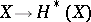from the category of varieties into the category of finite-dimensional graded anti-commutative-algebras, which satisfies the following conditions:

1) If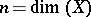, then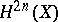is isomorphic to, and the mapping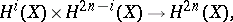defined by the multiplication in, is non-degenerate for all;

2)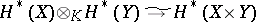(Künneth formula);

3) Mapping of cycles. There exists a functorial homomorphismfrom the group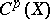of algebraic cycles inof codimensioninto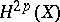which maps the direct product of cycles to the tensor product and is non-trivial in the sense that, for a point,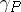becomes the canonical imbedding ofinto. The numberis known as the-th Betti number of the variety.

Examples. If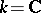, classical cohomology of complex manifolds with coefficients inis a Weil cohomology. Ifis a prime number distinct from the characteristic of the field, then étale-adic cohomology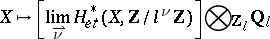is a Weil cohomology with coefficients in the field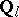.

The Lefschetz formula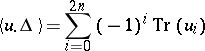is valid for Weil cohomology. In the above formula,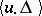is the intersection index inof the graphof the morphismwith the diagonal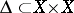, which may also be interpreted as the number of fixed points of the endomorphism, while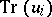is the trace of the endomorphismwhich is induced byin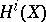. Moreover, this formula is also valid for correspondences, i.e. elements.